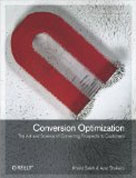• # Multiple Testing/Comparisons Problem: How Adding More Variations To Your AB Test Will Impact Your Results?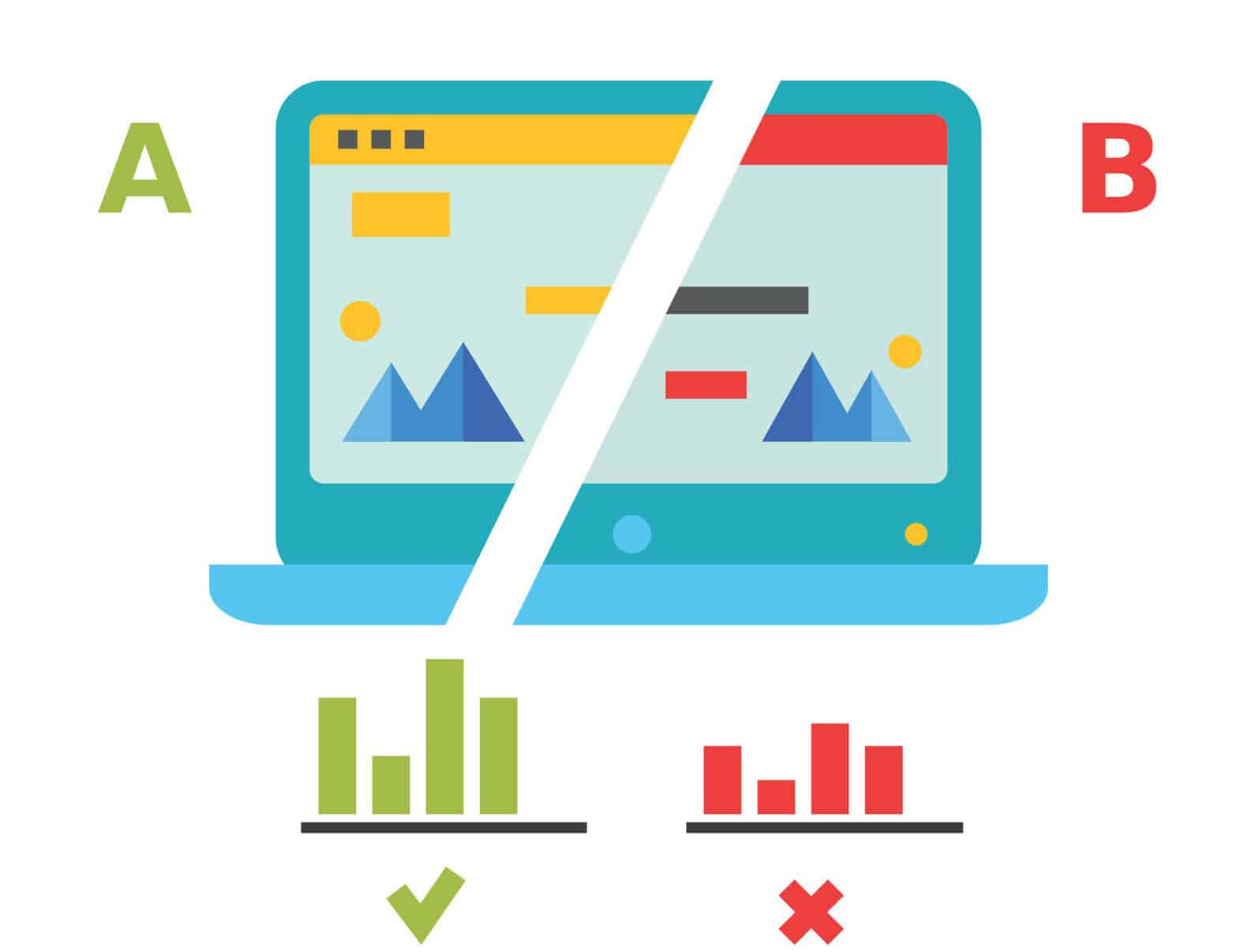I will take you back to 2007 to one of the very first CRO projects we conducted for a large online retailer. The website received a massive amount of traffic (100k per day). Since it was one of our first projects, and the team was a bit trigger happy with AB testing, our first test included 30 different designs to run against the control. The headache of implementing all of these designs was overwhelming. None the less, we launched the test after about six weeks of implementation.

Seven of our challengers beat the original design within five days. The team was ecstatic. We let the test run for a couple of more weeks, and the results remained consistent.

We finally stopped the test, selected one of the winning designs to be rolled out as the new design and started to monitor the site conversion rate.

It tanked. We tried another one of the winning designs. We did not see an uplift. We test each of the seven winning designs. In the best case scenario, the conversion rate remained the same. However, for the most part, these designs reduced the conversion rate.

There is a lot that we learned from that test regarding process and statistics. One of the early lessons that I will cover in this post is the number of variations we should test against the control in a split test.

Here statistics gives us a clear warning: the more variations (comparisons) you make, the higher the probability that you obtain a FALSE significant result.

There is always a chance of making a wrong decision when conducting an A/B test that has one challenger to the control. In this particular case, the test will have a single comparison between the control vs. the variation conversion rates.

When conducting a two-tailed test that compares two conversion rates of the control$\rho_{1}$ and the conversion rate for the variation ($\rho_{2}$), your hypothesis would be:

Null hypothesis:$H_{0}: \rho_{1}= \rho_{2}$
Alternative hypothesis:$H_{1} : \rho _{1} \neq \rho_{2}$

Your goal in conducting the AB test is to reject the null (H0) hypothesis that both rates are equal. You never accept the null hypothesis. If your test does not result in a winner, it means that you do not have enough evidence/data to reject the null hypothesis.

If the null hypothesis is true, so the two rates are equal, then you do not reject H0, and your decision is correct. The same applies when your test has a winner, which means that the null hypothesis (H0) is false and you reject it correctly.

However, when you reject a true null hypothesis (H0), you make a type I error (false positive). Similarly when the null hypothesis (H0) is false, but you fail to reject, then you make a type II error (false negative).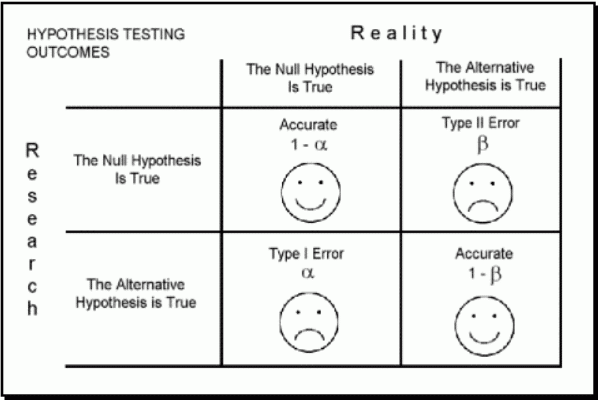## How to prevent these statistical errors when conducting an A/B test?

The probability of type II error (false negative) is denoted in statistics by beta. Increasing the sample size of your A/B test will prevent type II error from happening.

Alpha denotes the probability of type I error (false positive). You typically construct your test to keep it at a  significance level of 5% to minimize the possibility of type I errors.

The 5% significance level means that if you declare a winner in your test (reject the null hypothesis), then you have a 95% chance that you are correct in doing so. It also means that you have significant result difference between the control and the variation with a 95% “confidence.”

In reality, your A/B test will include multiple variations (or challengers) running against the control, which means you will have to run multiple comparisons (between the control conversion rate versus the different variation conversion rates).

##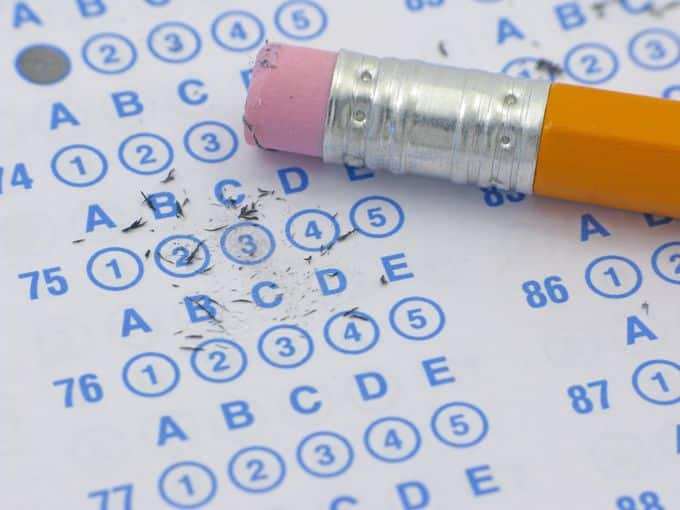With many comparisons, the probability of discovering AT LEAST ONE false significant result, i.e., incorrectly rejecting a null hypothesis, increases according to a formula:$P(TotalTypeIError)=(1- \alpha )^k,$

Where k is the number of variations in a test. So for a test that contains 10 different variations and a significance level of 5% (k equal 10 and alpha 0.05), the overall type I error increases to$(1-0.05)^{10}\approx0.40$.

This means that you have a 40% chance of finding a false negative: assuming that at least one of your variations is better [or worse] than the control when in fact it is not.

So, with ten variations your test has a 50% chance of getting a significant false result.

In the case, it’s better to toss a coin!

This is only for 10 comparisons of the control to each of the variations.

If you are running an A/B test with multiple variations against the control, then it is NOT enough to see if a variation is performing better than the control. You will also want to evaluate how each variation performs compared to the other variations so you can select the top performer for the test.  In the case, the chances of finding a false positive increase more and things get worse.

The graph below illustrates how the overall type I error increases as the number of tests increases.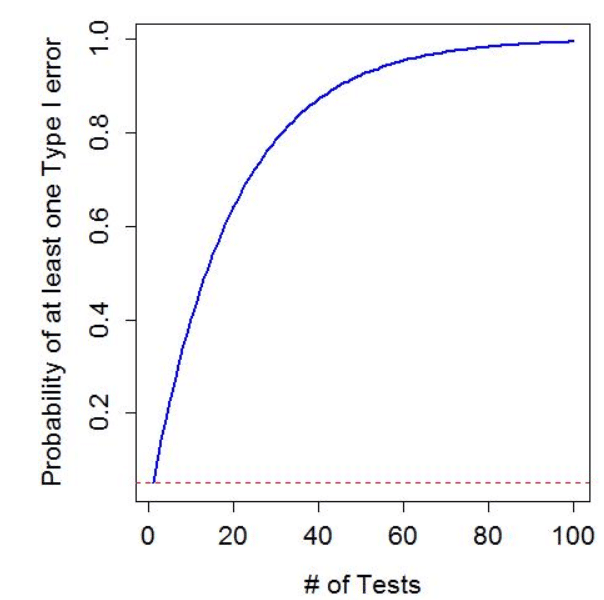The problem of increasing the chance of discovering a false positive as you increase the number of variations is ancient and well-known. It’s called multiple testing problem. According to University of California, Berkeley-Department of Statistics:

“In Statistics, multiple testing refers to the potential increase in Type I error that occurs when statistical tests are used repeatedly, for example while doing multiple comparisons to test null hypotheses stating that the averages of several disjoint populations are equal to each other (homogeneous).”

People struggle with this problem not only in A/B testing context but also in genetics. For example when you have millions of genetic variations to compare or in time series analysis when you would like to analyze a process over time and compare hundreds or thousands of different time points between each other.

## Possible solutions to multiple testing problemSo, how do you deal with the multiple testing problem?

The simplest solution that works for a limited number of comparisons is using statistical methods correction for multiple testing. These methods such as Bonferroni and Hochberg rely on some statistical adjustments made to p-values with the goal of reducing the chances of obtaining false-positive results. These methods are quite technical, so we won’t elaborate on the formulas used to derive them.

Both methods are based on the idea of adjusting significant levels for a single comparison. In Bonferroni correction for an A/B test, comparing three new designs to one control, the significance level for a single test would be 0.05 divided by 3 (number of variations). This simple method is often too conservative. In a sense, using the Bonferroni correction, we are more likely not to state any difference between the control and any other variation.

There are other, more complicated methods, some more or less conservative and people in many areas of science are still working to improve them.

## Using multivariate models to solve multiple testing problem

Another approach to solving the issue of multiple testing is through multivariate models. This is a conventional approach for time series analysis when you have an evolution of the process in time, and you look for some trend or evolution pattern.

In A/B testing, the variations usually cannot be measured in time. They do not have any structure unless they differ in some increasing feature that can be measured. However, this is very unlikely since nobody will increase a single button color on a page to construct such a series of variations. Each variation, in this case, should contain several changes as opposed to a single change. From a purely statistical analysis, these changes do not have any order. Even though you cannot order your variations, you can still model them in a multivariate way. However, the number of data per single variation must be reasonably high. Which means that you need to have enough traffic per each variation.

For A/B testing, one can go from straightforward methods like logistic models to more advanced ones such as neural networks. In which case,  you treat users as subjects and their behavior, i.e., whether a user bought a product or not as a binary 0/1 response. In such a scenario, the type of design is treated as a potential predictor in a model, and its significance is tested. The advantage of such an approach is the possibility of testing also other factors (such as the day of the week or type of the device the visitor is using) by including them in your model. Moreover, one can also add possible interactions between those factors and the nature of the design. For example, users might prefer design A over B but only if they use apple phone or just on the weekends.

One might use typical classification methods by treating the users’ behavior: bought (conversion) or not bought (no conversion) as two classes.

An example of such a tree is visible below: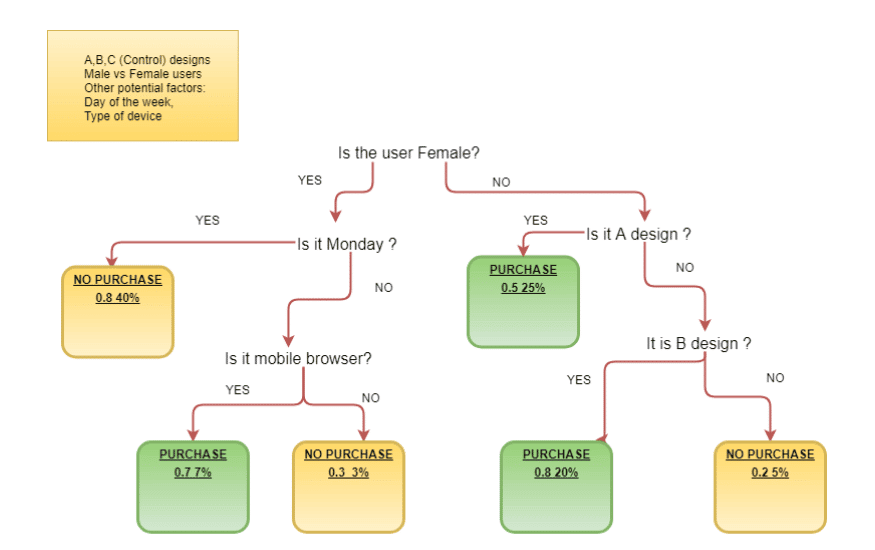The figures under the leaves show the probability of conversion and the percentage of observations (users) in the leaf. Looking at the numbers in the image above, we conclude the following:

1. For men, the essential factor that determines whether they purchase or not is the type of design.
2. For women, they are more likely to make purchases on other days than Monday using their mobile phone when it comes to a particular design.

The example illustrates, the more emotional shopping done by women and more rational by men 🙂

## Using More contrast (fewer variations) to solve multiple testing problem

The more contrast between the variations, the more chance for different users behavior and significant results. One of the more efficient solutions to deal with the multiple test problem is to create variations that are significantly different from each other and therefore can provide a significant test result.

To limit the number of tested factors, you should group the changes in a variation taking into account the nature of these changes, such as changes only in the page layout or changes in copy.

The more homogeneity within the groups and less between variation, the better your test is.

By having a small variability within the groups, you will be able to estimate the group effect. If the groups are polluted, and you have too much variability of user behavior, you will not be able to predict the conversion rate reliably. As a result, the differences between the tested groups will diminish.

##Each of the methods discussed above will help you limit the problem of multiple testing. However, they also require more statistical knowledge. An entirely different approach to the problem is to use bayesian testing to determine the winner in your test. According to Michael Frasco:

“Bayesian A/B testing accomplishes this without sacrificing reliability by controlling the magnitude of our bad decisions instead of the false positive rate.”

Summary

It is better to that your variations contain radical changes to the control. This is more time consuming but limits of the number of variations and the potential for multiple testing problem.

Small changes will not result in significant contrast and will cost you too much time, raising the risk of obtaining false results dramatically. At the same time, do not overdo it with changing too many factors at the same time! If you make many radical changes which result in determining a significant difference, you will not be able to conclude which factors caused the uplift.

Choose only a few critical factors and add them one by one or change them in some other logical way, so that later on you will be able to distinguish between the effect of a single factor and the impact of a group of factors.

# Khalid Saleh

Khalid Saleh is CEO and co-founder of Invesp. He is the co-author of Amazon.com bestselling book: "Conversion Optimization: The Art and Science of Converting Visitors into Customers." Khalid is an in-demand speaker who has presented at such industry events as SMX, SES, PubCon, Emetrics, ACCM and DMA, among others.

View All Posts By Khalid Saleh## Join 25,000+ Marketing Professionals

•